# Introduction to casebase sampling

## Methodological details

evaluate_vignette <- requireNamespace("eha", quietly = TRUE) &&
requireNamespace("visreg", quietly = TRUE) &&
requireNamespace("splines", quietly = TRUE)
knitr::opts_chunk$set(eval = evaluate_vignette) Case-base sampling was proposed by Hanley and Miettinen, 2009 as a way to fit smooth-in-time parametric hazard functions via logistic regression. The main idea, which was first proposed by Mantel, 1973 and then later developed by Efron, 1977, is to sample person-moments, i.e. discrete time points along an subject’s follow-up time, in order to construct a base series against which the case series can be compared. This approach allows the explicit inclusion of the time variable into the model, which enables the user to fit a wide class of parametric hazard functions. For example, including time linearly recovers the Gompertz hazard, whereas including time logarithmically recovers the Weibull hazard; not including time at all corresponds to the exponential hazard. The theoretical properties of this approach have been studied in Saarela and Arjas, 2015 and Saarela, 2015. ## Analysis of the veteran dataset The first example we discuss uses the well-known veteran dataset, which is part of the survival package. As we can see below, there is almost no censoring, and therefore we can get a good visual representation of the survival function: set.seed(12345) library(casebase) library(survival) data(veteran) table(veteran$status)
##
##   0   1
##   9 128
evtimes <- veteran$time[veteran$status == 1]
hist(evtimes, nclass = 30, main = '', xlab = 'Survival time (days)',
col = 'gray90', probability = TRUE)
tgrid <- seq(0, 1000, by = 10)
lines(tgrid, dexp(tgrid, rate = 1.0/mean(evtimes)),
lwd = 2, lty = 2, col = 'red')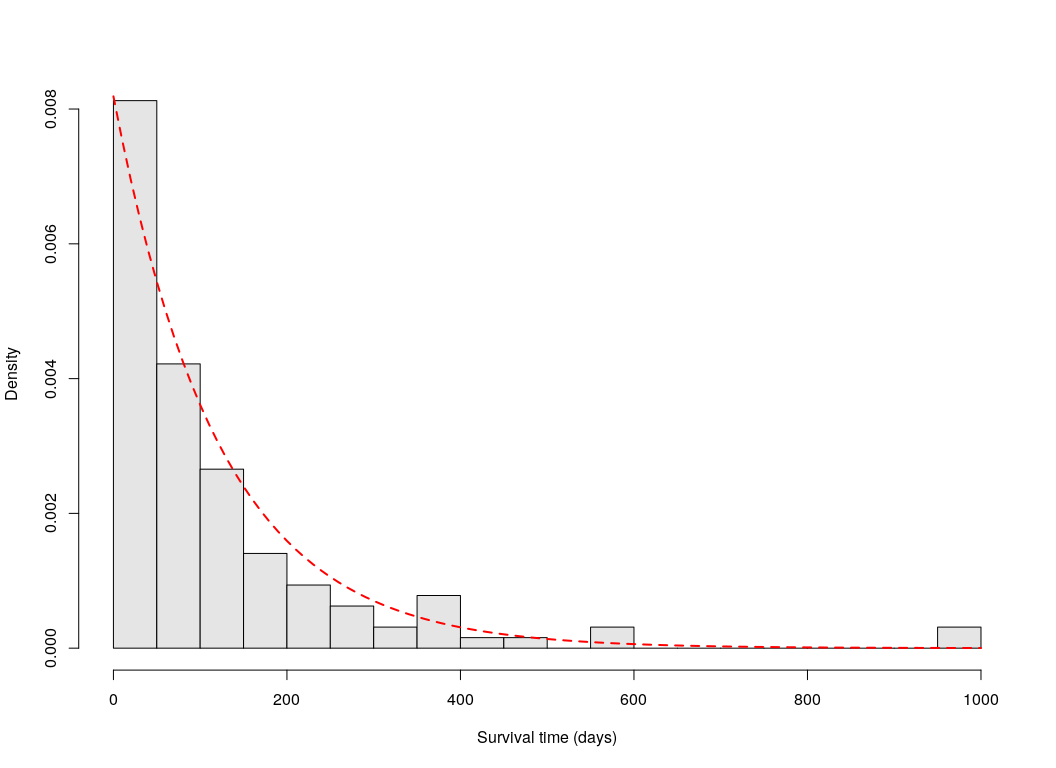As we can see, the empirical survival function resembles an exponential distribution.

We will first try to estimate the hazard function parametrically using some well-known regression routines. But first, we will reformat the data slightly.

veteran$prior <- factor(veteran$prior, levels = c(0, 10), labels = c("no","yes"))
veteran$celltype <- factor(veteran$celltype,
levels = c('large', 'squamous', 'smallcell', 'adeno'))
veteran$trt <- factor(veteran$trt, levels = c(1, 2), labels = c("standard", "test"))

Using the eha package, we can fit a Weibull form, with different values of the shape parameter. For shape = 1, we get an exponential distribution:

library(eha)
y <- with(veteran, Surv(time, status))

model1 <- weibreg(y ~ karno + diagtime + age + prior + celltype + trt,
data = veteran, shape = 1)
summary(model1)
## Call:
## weibreg(formula = y ~ karno + diagtime + age + prior + celltype +
##     trt, data = veteran, shape = 1)
##
## Covariate           Mean       Coef Exp(Coef)  se(Coef)    Wald p
## karno              68.419    -0.031     0.970     0.005     0.000
## diagtime            8.139     0.000     1.000     0.009     0.974
## age                57.379    -0.006     0.994     0.009     0.505
## prior
##               no    0.653     0         1           (reference)
##              yes    0.347     0.049     1.051     0.227     0.827
## celltype
##            large    0.269     0         1           (reference)
##         squamous    0.421    -0.377     0.686     0.273     0.166
##        smallcell    0.206     0.443     1.557     0.261     0.090
##            adeno    0.104     0.736     2.087     0.294     0.012
## trt
##         standard    0.477     0         1           (reference)
##             test    0.523     0.220     1.246     0.199     0.269
##
## log(scale)                    2.811    16.633     0.713     0.000
##
##  Shape is fixed at  1
##
## Events                    128
## Total time at risk         16663
## Max. log. likelihood      -716.16
## LR test statistic         70.1
## Degrees of freedom        8
## Overall p-value           4.64229e-12

If we take shape = 0, the shape parameter is estimated along with the regression coefficients:

model2 <- weibreg(y ~ karno + diagtime + age + prior + celltype + trt,
data = veteran, shape = 0)
summary(model2)
## Call:
## weibreg(formula = y ~ karno + diagtime + age + prior + celltype +
##     trt, data = veteran, shape = 0)
##
## Covariate           Mean       Coef Exp(Coef)  se(Coef)    Wald p
## karno              68.419    -0.032     0.968     0.005     0.000
## diagtime            8.139     0.001     1.001     0.009     0.955
## age                57.379    -0.007     0.993     0.009     0.476
## prior
##               no    0.653     0         1           (reference)
##              yes    0.347     0.047     1.048     0.229     0.836
## celltype
##            large    0.269     0         1           (reference)
##         squamous    0.421    -0.428     0.651     0.278     0.123
##        smallcell    0.206     0.462     1.587     0.262     0.078
##            adeno    0.104     0.792     2.208     0.300     0.008
## trt
##         standard    0.477     0         1           (reference)
##             test    0.523     0.246     1.279     0.203     0.224
##
## log(scale)                    2.864    17.537     0.671     0.000
## log(shape)                    0.075     1.077     0.066     0.261
##
## Events                    128
## Total time at risk         16663
## Max. log. likelihood      -715.55
## LR test statistic         65.1
## Degrees of freedom        8
## Overall p-value           4.65393e-11

Finally, we can also fit a Cox proportional hazard:

model3 <- coxph(y ~ karno + diagtime + age + prior + celltype + trt,
data = veteran)
summary(model3)
## Call:
## coxph(formula = y ~ karno + diagtime + age + prior + celltype +
##     trt, data = veteran)
##
##   n= 137, number of events= 128
##
##                         coef  exp(coef)   se(coef)      z Pr(>|z|)
## karno             -3.282e-02  9.677e-01  5.508e-03 -5.958 2.55e-09 ***
## diagtime           8.132e-05  1.000e+00  9.136e-03  0.009  0.99290
## age               -8.706e-03  9.913e-01  9.300e-03 -0.936  0.34920
## prioryes           7.159e-02  1.074e+00  2.323e-01  0.308  0.75794
## celltypesquamous  -4.013e-01  6.695e-01  2.827e-01 -1.420  0.15574
## celltypesmallcell  4.603e-01  1.584e+00  2.662e-01  1.729  0.08383 .
## celltypeadeno      7.948e-01  2.214e+00  3.029e-01  2.624  0.00869 **
## trttest            2.946e-01  1.343e+00  2.075e-01  1.419  0.15577
## ---
## Signif. codes:  0 '***' 0.001 '**' 0.01 '*' 0.05 '.' 0.1 ' ' 1
##
##                   exp(coef) exp(-coef) lower .95 upper .95
## karno                0.9677     1.0334    0.9573    0.9782
## diagtime             1.0001     0.9999    0.9823    1.0182
## age                  0.9913     1.0087    0.9734    1.0096
## prioryes             1.0742     0.9309    0.6813    1.6937
## celltypesquamous     0.6695     1.4938    0.3847    1.1651
## celltypesmallcell    1.5845     0.6311    0.9403    2.6699
## celltypeadeno        2.2139     0.4517    1.2228    4.0084
## trttest              1.3426     0.7448    0.8939    2.0166
##
## Concordance= 0.736  (se = 0.021 )
## Likelihood ratio test= 62.1  on 8 df,   p=2e-10
## Wald test            = 62.37  on 8 df,   p=2e-10
## Score (logrank) test = 66.74  on 8 df,   p=2e-11

As we can see, all three models are significant, and they give similar information: karno and celltype are significant predictors, both treatment is not.

The method available in this package makes use of case-base sampling. That is, person-moments are randomly sampled across the entire follow-up time, with some moments corresponding to cases and others to controls. By sampling person-moments instead of individuals, we can then use logistic regression to fit smooth-in-time parametric hazard functions. See the previous section for more details.

First, we will look at the follow-up time by using population-time plots:

# create popTime object
pt_veteran <- popTime(data = veteran)
## 'time' will be used as the time variable
## 'status' will be used as the event variable
class(pt_veteran)
##  "popTime"    "data.table" "data.frame"
# plot method for objects of class 'popTime'
plot(pt_veteran)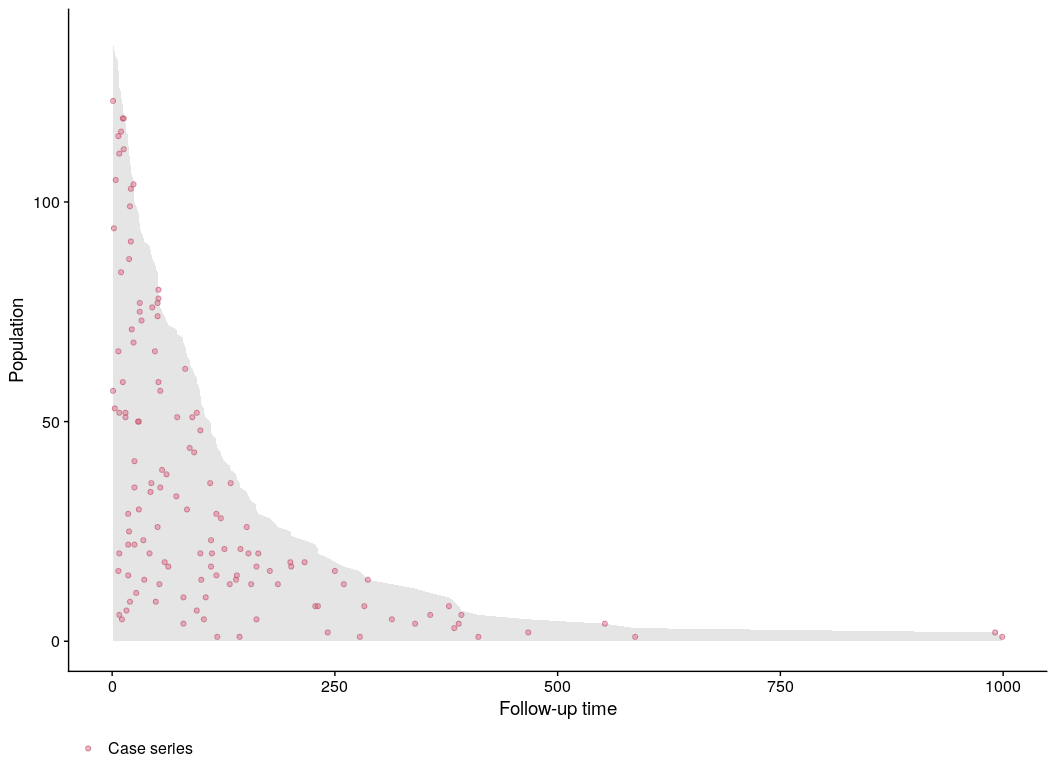Population-time plots are a useful way of visualizing the total follow-up experience, where individuals appear on the y-axis, and follow-up time on the x-axis; each individual’s follow-up time is represented by a gray line segment. For convenience, we have ordered the patients according to their time-to-event, and each event is represented by a red dot. The censored observations (of which there is only a few) correspond to the grey lines which do not end with a red dot.

Next, we use case-base sampling to fit a parametric hazard function via logistic regression. First, we will include time as a linear term; as noted above, this corresponds to an Gompertz hazard.

model4 <- fitSmoothHazard(status ~ time + karno + diagtime + age + prior +
celltype + trt, data = veteran, ratio = 100)
## 'time' will be used as the time variable
summary(model4)
##
## Call:
## glm(formula = formula, family = binomial, data = sampleData)
##
## Deviance Residuals:
##     Min       1Q   Median       3Q      Max
## -0.4285  -0.1501  -0.1199  -0.1002   3.4177
##
## Coefficients:
##                     Estimate Std. Error z value Pr(>|z|)
## (Intercept)       -2.6888658  0.7213316  -3.728 0.000193 ***
## time               0.0003337  0.0006450   0.517 0.604898
## karno             -0.0324322  0.0052911  -6.130 8.81e-10 ***
## diagtime           0.0035611  0.0093088   0.383 0.702056
## age               -0.0066025  0.0092944  -0.710 0.477471
## prioryes           0.0089338  0.2313008   0.039 0.969190
## celltypesquamous  -0.4319329  0.2844682  -1.518 0.128917
## celltypesmallcell  0.3941374  0.2626738   1.500 0.133489
## celltypeadeno      0.7020877  0.2987435   2.350 0.018767 *
## trttest            0.2109057  0.2018479   1.045 0.296081
## ---
## Signif. codes:  0 '***' 0.001 '**' 0.01 '*' 0.05 '.' 0.1 ' ' 1
##
## (Dispersion parameter for binomial family taken to be 1)
##
##     Null deviance: 1436.2  on 12927  degrees of freedom
## Residual deviance: 1365.7  on 12918  degrees of freedom
## AIC: 1385.7
##
## Number of Fisher Scoring iterations: 8

Since the output object from fitSmoothHazard inherits from the glm class, we see a familiar result when using the function summary. We can quickly visualize the conditional association between each predictor and the hazard function using the plot method for objects that are fit with fitSmoothHazard. Specifically, if $$x$$ is the predictor of interest, $$h$$ is the hazard function, and $$\mathbf{x_{-j}}$$ the other predictors in the model, the conditional association plot represents the relationship $$f(x) = \mathbb{E}(h|x, \mathbf{x_{-j}})$$. By default, the other terms in the model ($$\mathbf{x_{-j}}$$) are set to their median if the term is numeric or the most common category if the term is a factor. Further details of customizing these plots are given in the Plot Hazards and Hazard Ratios vignette.

library(visreg)
plot(model4,
hazard.params = list(alpha = 0.05))
## Conditions used in construction of plot
## karno: 70
## diagtime: 5
## age: 60
## prior: no
## celltype: squamous
## trt: test
## offset: 0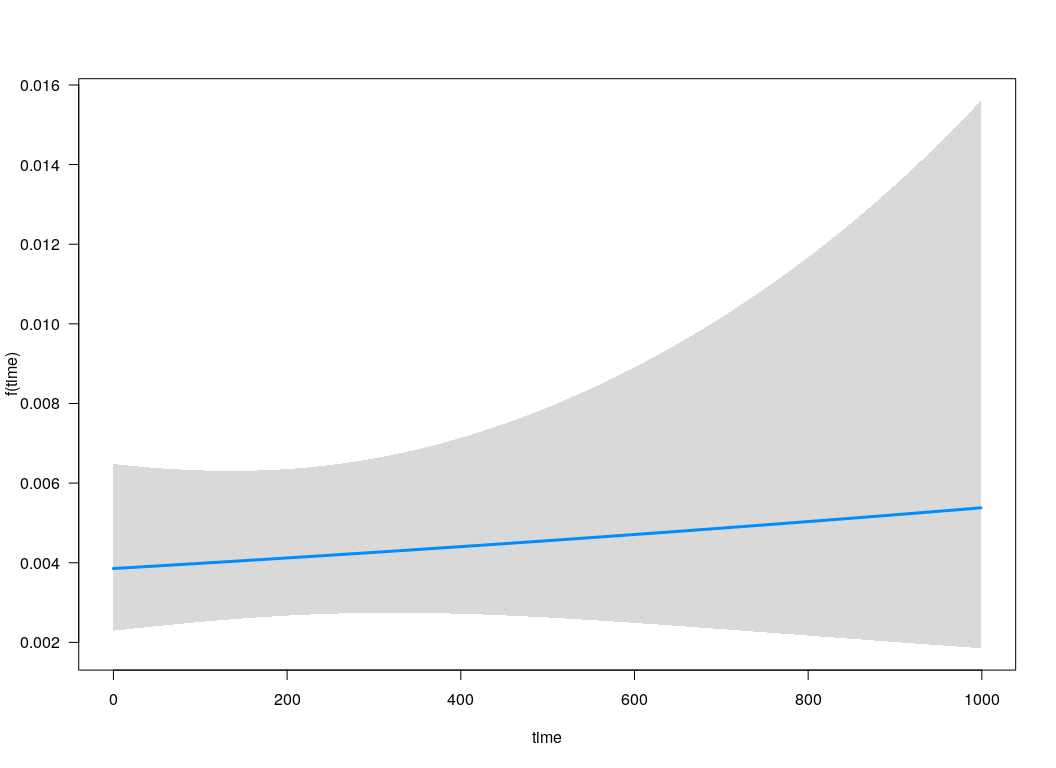## Conditions used in construction of plot
## time: 93.41294
## diagtime: 5
## age: 60
## prior: no
## celltype: squamous
## trt: test
## offset: 0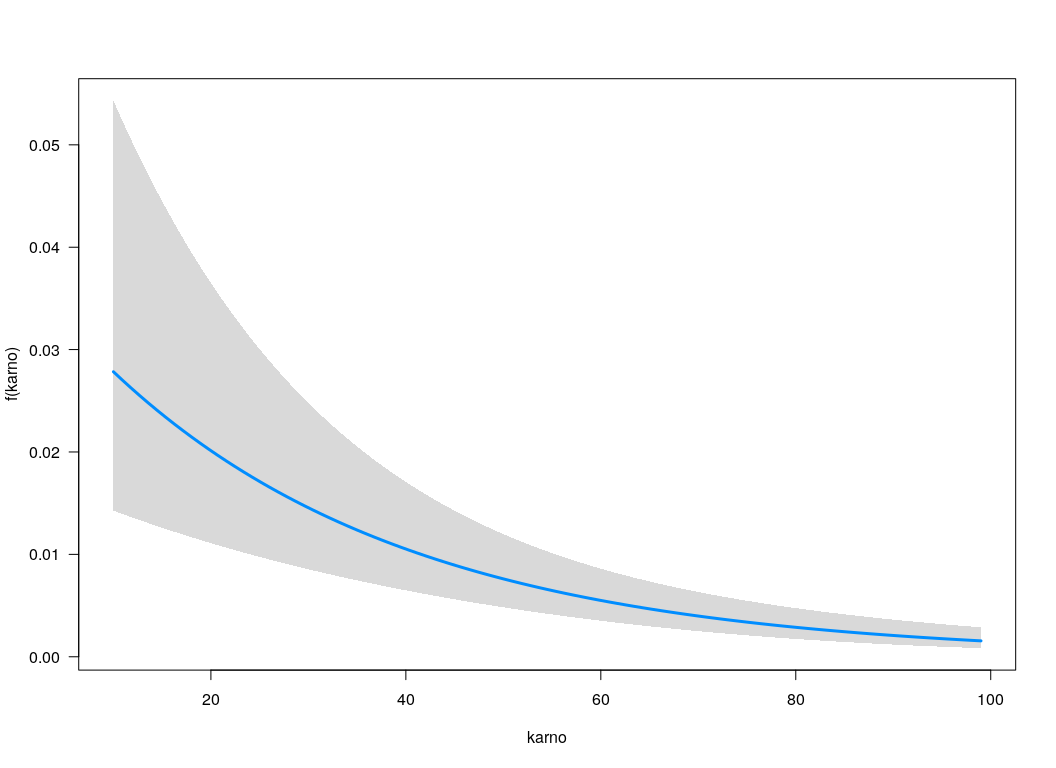## Conditions used in construction of plot
## time: 93.41294
## karno: 70
## age: 60
## prior: no
## celltype: squamous
## trt: test
## offset: 0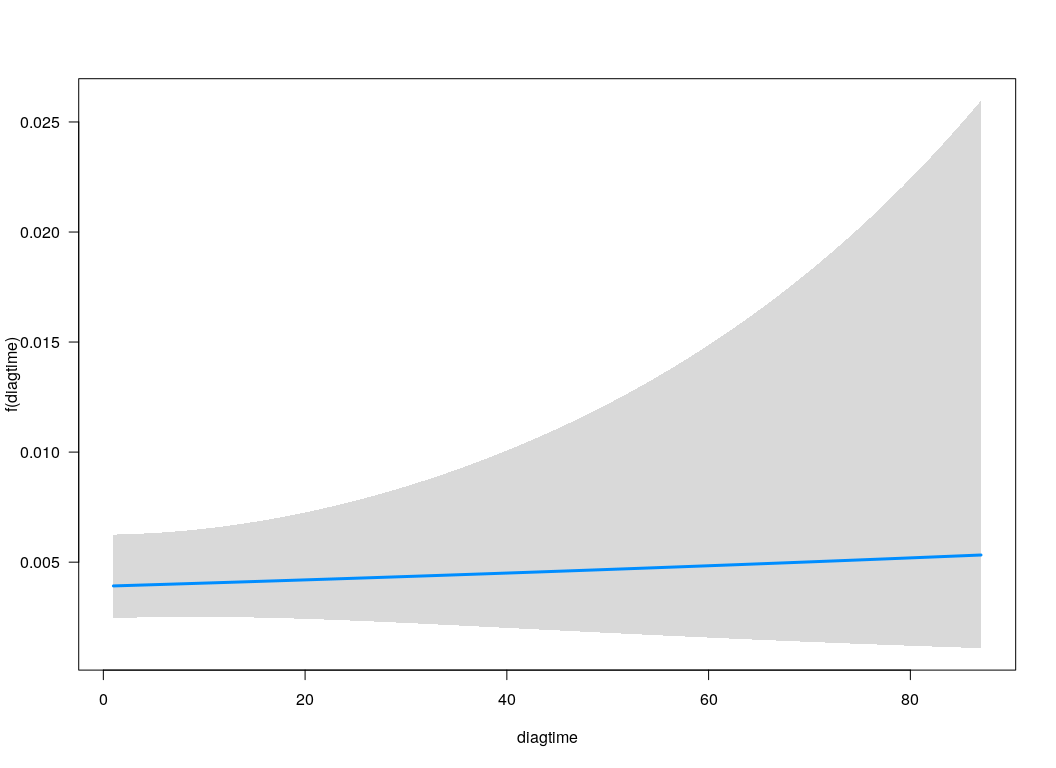## Conditions used in construction of plot
## time: 93.41294
## karno: 70
## diagtime: 5
## prior: no
## celltype: squamous
## trt: test
## offset: 0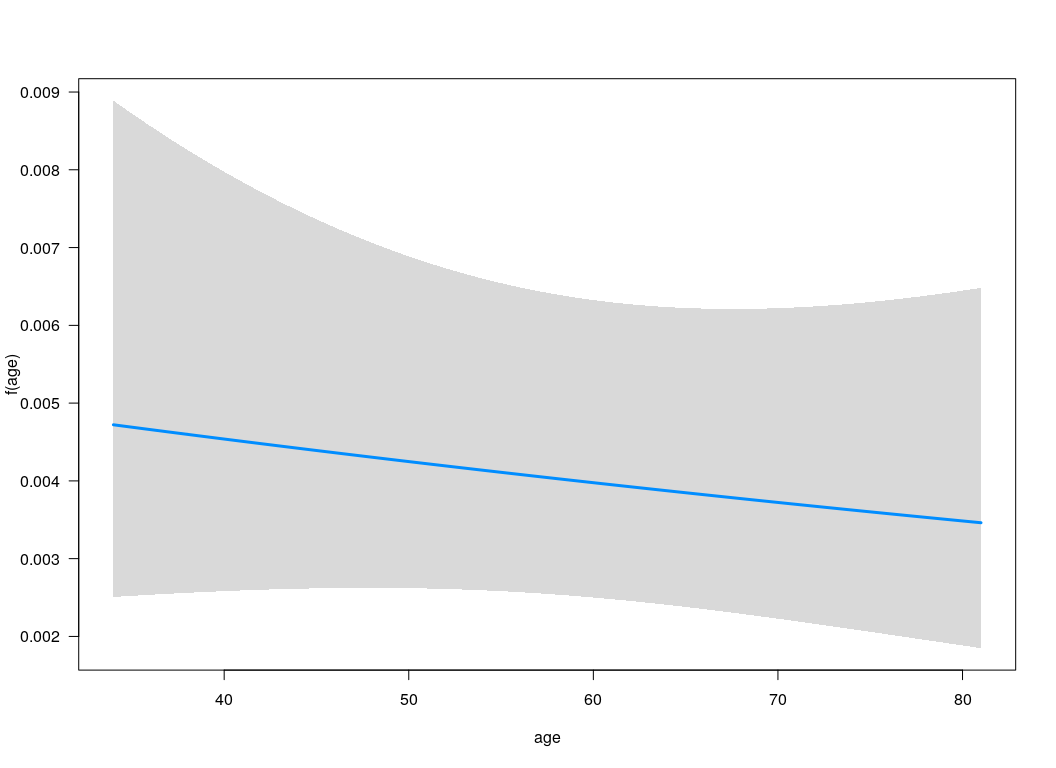## Conditions used in construction of plot
## time: 93.41294
## karno: 70
## diagtime: 5
## age: 60
## celltype: squamous
## trt: test
## offset: 0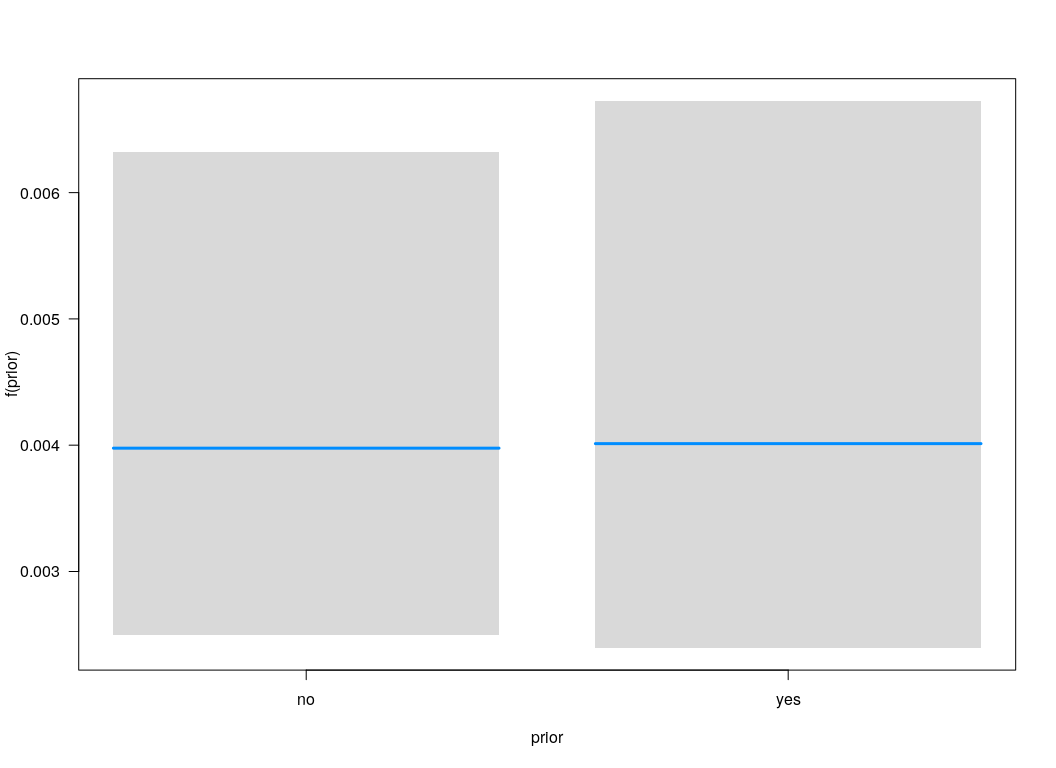## Conditions used in construction of plot
## time: 93.41294
## karno: 70
## diagtime: 5
## age: 60
## prior: no
## trt: test
## offset: 0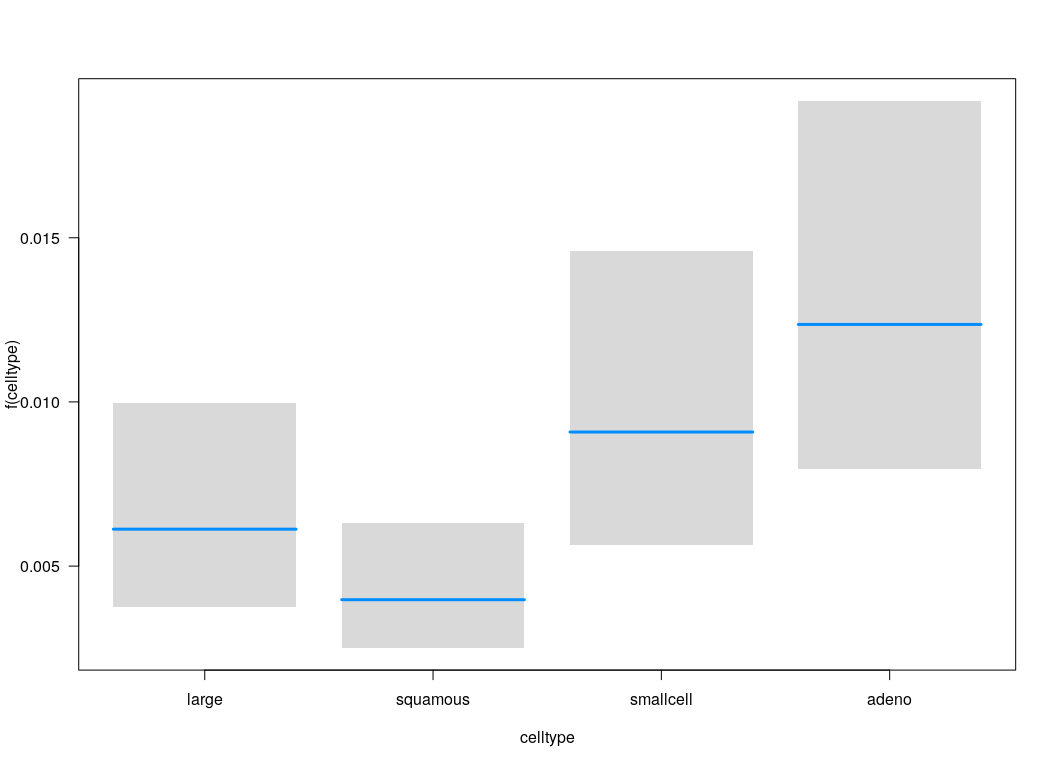## Conditions used in construction of plot
## time: 93.41294
## karno: 70
## diagtime: 5
## age: 60
## prior: no
## celltype: squamous
## offset: 0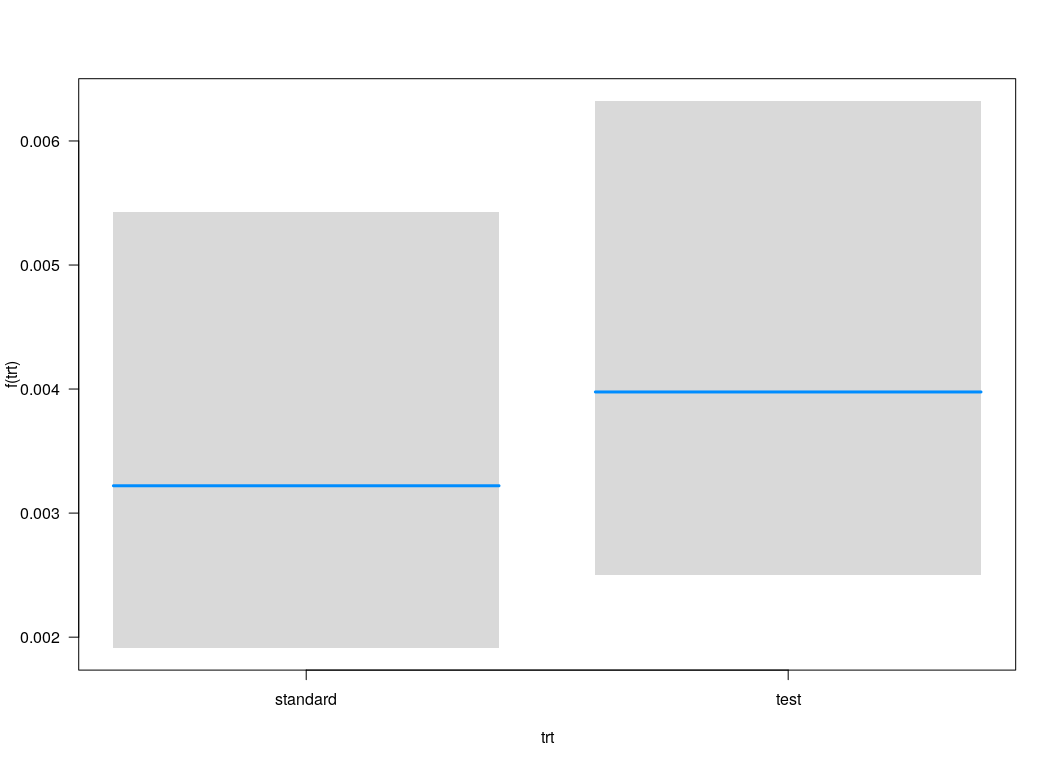## []
## $fit ## time karno diagtime age prior celltype trt offset status visregFit ## visregLwr visregUpr ## [ reached 'max' / getOption("max.print") -- omitted 101 rows ] ## ## [ reached getOption("max.print") -- omitted 2 entries ] ## attr(,"class") ##  "visreg" ## ## [ reached getOption("max.print") -- omitted 6 entries ] ## attr(,"class") ##  "visregList" The main purpose of fitting smooth hazard functions is that it is then relatively easy to compute absolute risks. For example, we can use the function absoluteRisk to compute the mean absolute risk at 90 days, which can then be compared to the empirical measure. absRisk4 <- absoluteRisk(object = model4, time = 90) mean(absRisk4) ##  0.6124916 ftime <- veteran$time
mean(ftime <= 90)
##  0.5547445

We can also fit a Weibull hazard by using a logarithmic term for time:

model5 <- fitSmoothHazard(status ~ log(time) + karno + diagtime + age + prior +
celltype + trt, data = veteran, ratio = 100)
## 'time' will be used as the time variable
summary(model5)
##
## Call:
## glm(formula = formula, family = binomial, data = sampleData)
##
## Deviance Residuals:
##     Min       1Q   Median       3Q      Max
## -0.4637  -0.1518  -0.1190  -0.0967   3.4205
##
## Coefficients:
##                     Estimate Std. Error z value Pr(>|z|)
## (Intercept)       -3.0686578  0.7552335  -4.063 4.84e-05 ***
## log(time)          0.0721936  0.0718563   1.005   0.3150
## karno             -0.0328926  0.0055067  -5.973 2.33e-09 ***
## diagtime          -0.0007996  0.0091842  -0.087   0.9306
## age               -0.0045541  0.0092918  -0.490   0.6240
## prioryes           0.0286398  0.2292875   0.125   0.9006
## celltypesquamous  -0.4170963  0.2799527  -1.490   0.1363
## celltypesmallcell  0.4495348  0.2634379   1.706   0.0879 .
## celltypeadeno      0.7776537  0.3036331   2.561   0.0104 *
## trttest            0.2740183  0.2046518   1.339   0.1806
## ---
## Signif. codes:  0 '***' 0.001 '**' 0.01 '*' 0.05 '.' 0.1 ' ' 1
##
## (Dispersion parameter for binomial family taken to be 1)
##
##     Null deviance: 1436.2  on 12927  degrees of freedom
## Residual deviance: 1365.1  on 12918  degrees of freedom
## AIC: 1385.1
##
## Number of Fisher Scoring iterations: 8

With case-base sampling, it is straightforward to fit a semi-parametric hazard function using splines, which can then be used to estimate the mean absolute risk.

# Fit a spline for time
library(splines)
model6 <- fitSmoothHazard(status ~ bs(time) + karno + diagtime + age + prior +
celltype + trt, data = veteran, ratio = 100)
## 'time' will be used as the time variable
summary(model6)
##
## Call:
## glm(formula = formula, family = binomial, data = sampleData)
##
## Deviance Residuals:
##     Min       1Q   Median       3Q      Max
## -0.4551  -0.1535  -0.1198  -0.0952   3.5218
##
## Coefficients:
##                     Estimate Std. Error z value Pr(>|z|)
## (Intercept)       -2.9343444  0.7277306  -4.032 5.53e-05 ***
## bs(time)1          1.6365070  1.0324136   1.585  0.11294
## bs(time)2         -2.5135557  1.7558703  -1.432  0.15228
## bs(time)3          1.6976154  0.9897559   1.715  0.08631 .
## karno             -0.0322573  0.0053904  -5.984 2.17e-09 ***
## diagtime           0.0003886  0.0091607   0.042  0.96617
## age               -0.0065366  0.0093554  -0.699  0.48474
## prioryes           0.0162450  0.2356069   0.069  0.94503
## celltypesquamous  -0.4172317  0.2837950  -1.470  0.14151
## celltypesmallcell  0.4518275  0.2651004   1.704  0.08831 .
## celltypeadeno      0.8527251  0.3040353   2.805  0.00504 **
## trttest            0.2622058  0.2073319   1.265  0.20599
## ---
## Signif. codes:  0 '***' 0.001 '**' 0.01 '*' 0.05 '.' 0.1 ' ' 1
##
## (Dispersion parameter for binomial family taken to be 1)
##
##     Null deviance: 1436.2  on 12927  degrees of freedom
## Residual deviance: 1363.0  on 12916  degrees of freedom
## AIC: 1387
##
## Number of Fisher Scoring iterations: 8
str(absoluteRisk(object = model6, time = 90))
##  'absRiskCB' num [1:2, 1:138] 0 90 0 0.294 0 ...
##  - attr(*, "dimnames")=List of 2
##   ..$: chr [1:2] "" "" ## ..$ : chr [1:138] "time" "" "" "" ...
##  - attr(*, "type")= chr "CI"

As we can see from the summary, there is little evidence that splines actually improve the fit. Moreover, we can see that estimated individual absolute risks are essentially the same when using either a linear term or splines:

linearRisk <- absoluteRisk(object = model4, time = 90, newdata = veteran)
splineRisk <- absoluteRisk(object = model6, time = 90, newdata = veteran)

plot.default(linearRisk, splineRisk,
xlab = "Linear", ylab = "Splines", pch = 19)
abline(a = 0, b = 1, lty = 2, lwd = 2, col = 'red')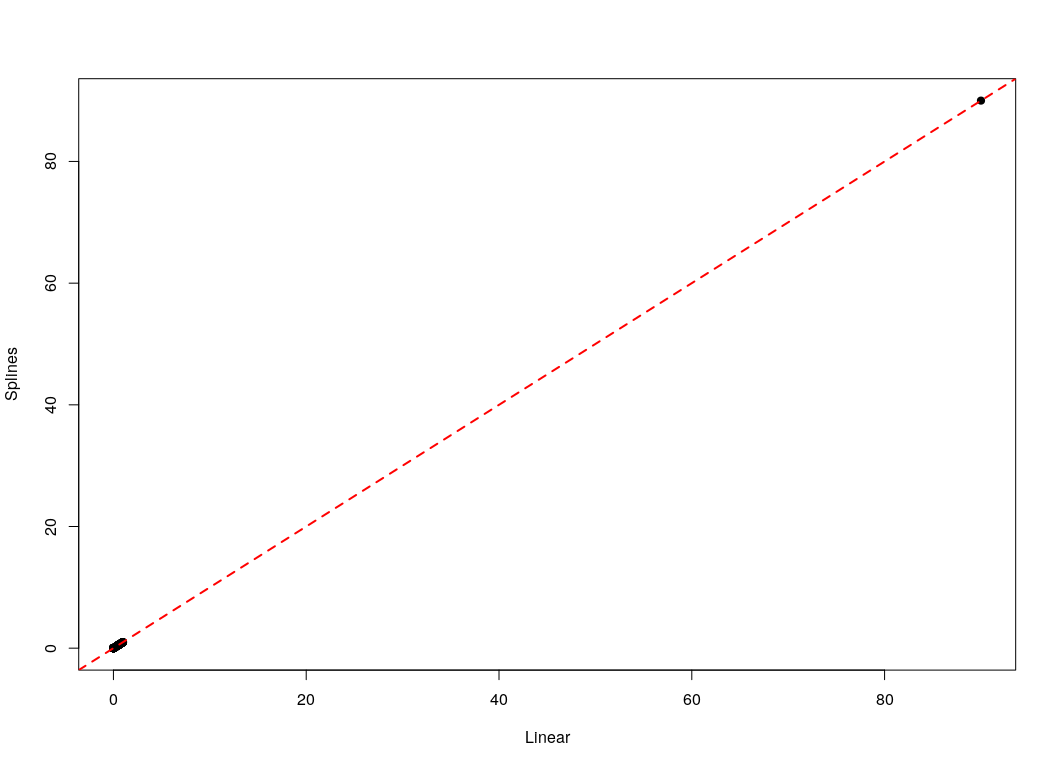These last three models give similar information as the first three, i.e. the main predictors for the hazard are karno and celltype, with treatment being non-significant. Moreover, by explicitely including the time variable in the formula, we see that it is not significant; this is evidence that the true hazard is exponential.

Finally, we can look at the estimates of the coefficients for the Cox model, as well as the last three models (CB stands for “case-base”):

Cox model CB linear CB log-linear CB splines
karno -0.0328 -0.0324 -0.0329 -0.0323
diagtime 0.0001 0.0036 -0.0008 0.0004
age -0.0087 -0.0066 -0.0046 -0.0065
prioryes 0.0716 0.0089 0.0286 0.0162
celltypesquamous -0.4013 -0.4319 -0.4171 -0.4172
celltypesmallcell 0.4603 0.3941 0.4495 0.4518
celltypeadeno 0.7948 0.7021 0.7777 0.8527
trttest 0.2946 0.2109 0.2740 0.2622

## Cumulative Incidence Curves

Here we show how to calculate the cumulative incidence curves for a specific risk profile using the following equation:

$CI(x, t) = 1 - exp\left[ - \int_0^t h(x, u) \textrm{d}u \right]$ where $$h(x, t)$$ is the hazard function, $$t$$ denotes the numerical value (number of units) of a point in prognostic/prospective time and $$x$$ is the realization of the vector $$X$$ of variates based on the patient’s profile and intervention (if any).

We compare the cumulative incidence functions from the fully-parametric fit using case base sampling, with those from the Cox model:

# define a specific covariate profile
new_data <- data.frame(trt = "test",
karno = median(veteran$karno), diagtime = median(veteran$diagtime),
age = median(veteran$age), prior = "no") # calculate cumulative incidence using casebase model smooth_risk <- absoluteRisk(object = model4, time = seq(0,300, 1), newdata = new_data) cols <- c("#8E063B","#023FA5") # cumulative incidence function for the Cox model plot(survfit(model3, newdata = new_data), xlab = "Days", ylab = "Cumulative Incidence (%)", fun = "event", xlim = c(0,300), conf.int = F, col = cols, main = sprintf("Estimated Cumulative Incidence (risk) of Lung Cancer\ntrt = test, celltype = adeno, karno = %g,\ndiagtime = %g, age = %g, prior = no", median(veteran$karno), median(veteran$diagtime), median(veteran$age)))

# add casebase curve with legend
plot(smooth_risk, add = TRUE, col = cols, gg = FALSE)
legend("bottomright",
legend = c("semi-parametric (Cox)", "parametric (casebase)"),
col = cols,
lty = c(1, 1),
bg = "gray90")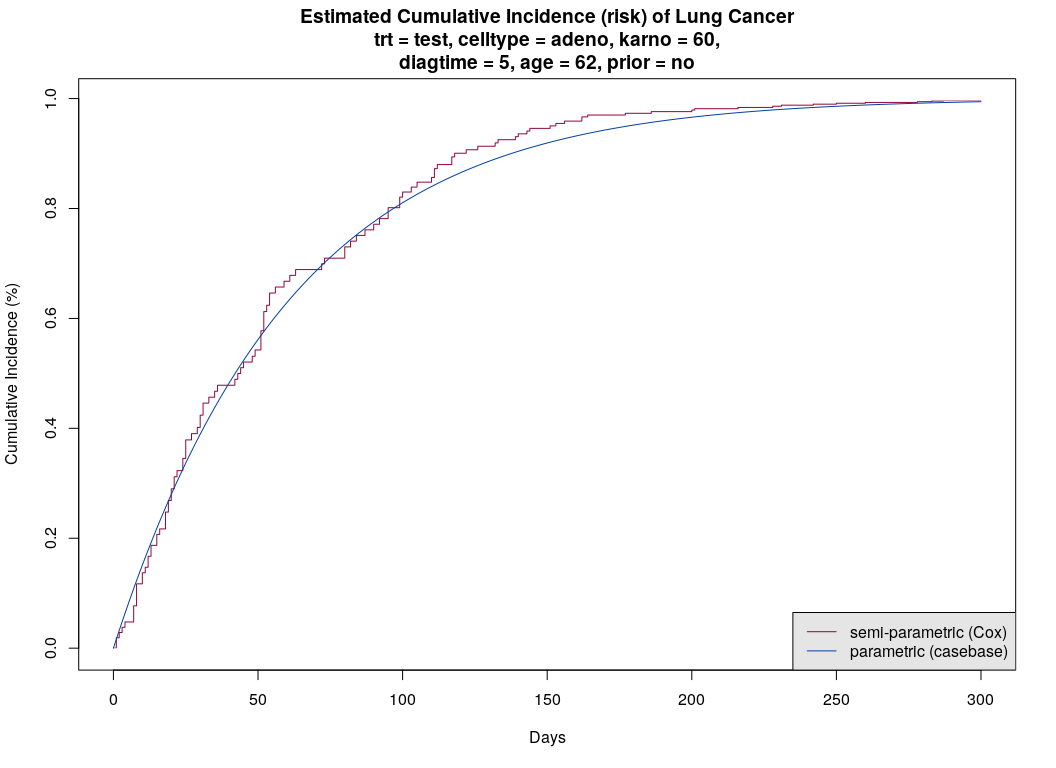Note that by default, absoulteRisk calculated the cumulative incidence. Alternatively, you can calculate the survival curve by specifying type = 'survival' in the call to absoulteRisk:

smooth_risk <- absoluteRisk(object = model4,
time = seq(0,300, 1),
newdata = new_data,
type = "survival")

plot(survfit(model3, newdata = new_data),
xlab = "Days", ylab = "Survival Probability (%)",
xlim = c(0,300), conf.int = F, col = cols,
main = sprintf("Estimated Survival Probability of Lung Cancer\ntrt = test, celltype = adeno, karno = %g,\ndiagtime = %g, age = %g, prior = no", median(veteran$karno), median(veteran$diagtime),
median(veteran\$age)))

# add casebase curve with legend
plot(smooth_risk, add = TRUE, col = cols, gg = FALSE)
legend("topright",
legend = c("semi-parametric (Cox)", "parametric (casebase)"),
col = cols,
lty = c(1, 1),
bg = "gray90")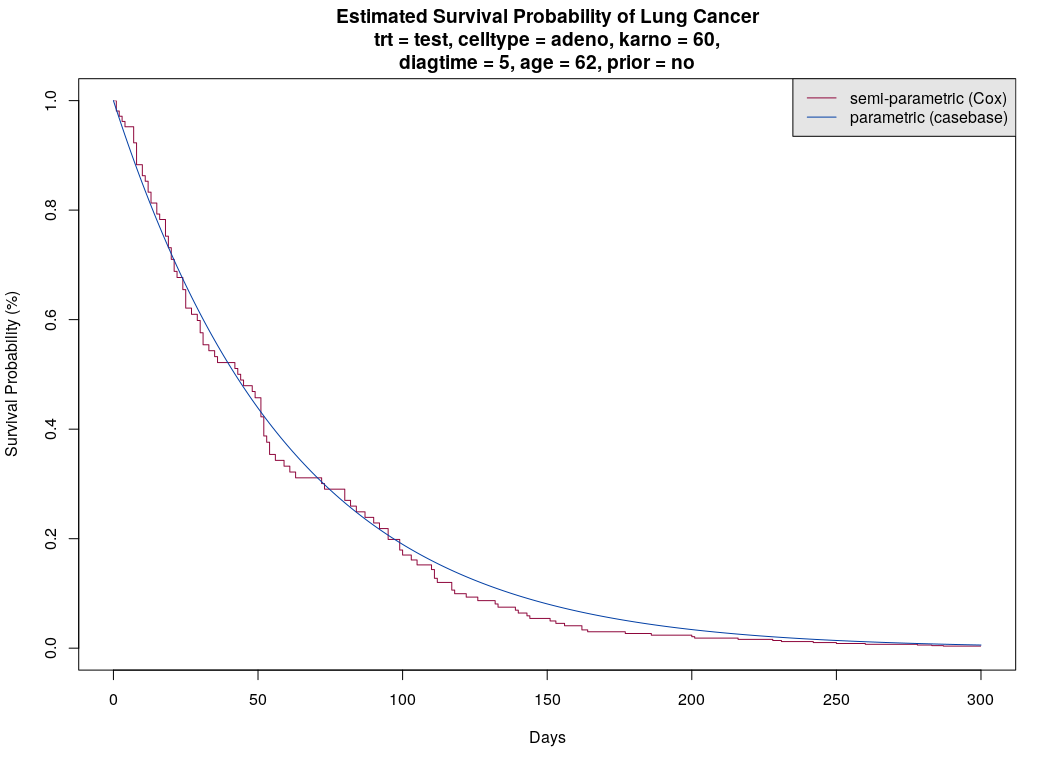## Session information

## R version 3.6.2 (2019-12-12)
## Platform: x86_64-pc-linux-gnu (64-bit)
## Running under: Pop!_OS 19.10
##
## Matrix products: default
## BLAS:   /usr/lib/x86_64-linux-gnu/openblas/libblas.so.3
## LAPACK: /usr/lib/x86_64-linux-gnu/libopenblasp-r0.3.7.so
##
## attached base packages:
##  splines   stats     graphics  grDevices utils     datasets  methods
##  base
##
## other attached packages:
##  eha_2.8.1          visreg_2.7.0       colorspace_1.4-1   data.table_1.12.8
##  ggplot2_3.3.2.9000 survival_3.1-8     casebase_0.9.0
##
## loaded via a namespace (and not attached):
##   Rcpp_1.0.4.6     highr_0.8        pillar_1.4.4     compiler_3.6.2
##   iterators_1.0.12 tools_3.6.2      digest_0.6.25    evaluate_0.14
##   lifecycle_0.2.0  tibble_3.0.1     gtable_0.3.0     nlme_3.1-143
##  lattice_0.20-38  mgcv_1.8-31      pkgconfig_2.0.3  rlang_0.4.6
##  foreach_1.5.0    Matrix_1.2-18    yaml_2.2.1       xfun_0.15
##  withr_2.2.0      stringr_1.4.0    dplyr_0.8.5      knitr_1.29
##  vctrs_0.3.1      glmnet_4.0-2     stats4_3.6.2     grid_3.6.2
##  tidyselect_1.0.0 glue_1.4.1       R6_2.4.1         VGAM_1.1-3
##  rmarkdown_2.3    farver_2.0.3     purrr_0.3.3      magrittr_1.5
##  codetools_0.2-16 scales_1.1.1     ellipsis_0.3.1   htmltools_0.5.0
##  assertthat_0.2.1 shape_1.4.4      labeling_0.3     stringi_1.4.6
##  munsell_0.5.0    crayon_1.3.4